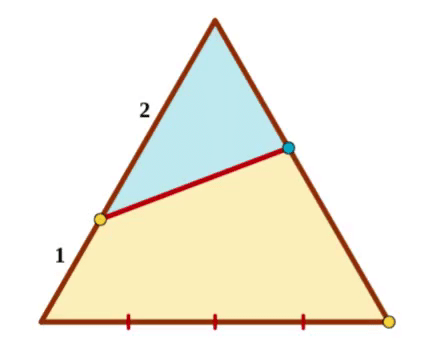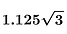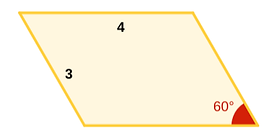4 Vertices, 4 Midpoints

The blue segments connect a vertex to the midpoint of a side. What fraction of the square's area is represented by the yellow quadrilateral?

Solution:

1. Find the equations for the 2 blue sides of the blue triangle. The x-coordinate of their intersection point will give us the height of the triangle so we can find its area:

2. We have a total of 4 triangles of the same size as the blue triangle, therefore the yellow area is:Concentric Circles

The length of the red segment is 2. What is the area of the blue ring?pi
2 pi
4 pi
0.5 pi

Solution:3 Vertices, 2 Midpoints

The blue segments connect a vertex to the midpoint of a side. What fraction of the square's area is represented by the yellow quadrilateral?

Solution:

1. The yellow area together with the triangles on its right and left represent a quarter of the square's area.

2. Find the equation for the 3 segments. Their intersection points help us find the heights of the two triangles.Triangle + Segment

Can you bisect a segment using only an unmarked ruler and a pattern in the shape of a scalene triangle?

Solution:

1. Use the pattern to draw two copies of the triangle, one at each end of the segment.

2. Connect the outer vertices of the triangles.

3. The two yellow triangles are congruent, therefore the two sub-parts of the green segment are congruent and the red point is its midpoint.Let's Reduce!

How many of the following 150 fractions can be reduced?

Solution:

1. For any fraction, if there is a number that divides into both its numerator and its denominator, then that number also divides into their sum.

2. The pattern in the sequence above is such that for any given fraction the numerator and the denominator add up to 151, a prime number.

3. Since there is no number (other than 1) that divides into 151, there is also no number that divides into both the numerator and the denominator of any of the given fractions. So no, none of them are reducible!Compass + Triangle

The base of the equilateral triangle is dissected into four equal parts.

Using only a compass, can you place the blue point such that the yellow and the blue areas are equal?

Solution:

1. The area of an equilateral triangle of side length 3 is:

2. The area of the blue triangle has to be half the area of the equilateral triangle:

3. Draw the altitude from the blue vertex to the side of length 2 to create a 30-60-90 special right triangle.

4. Set the blue area (in terms of x) equal to the value found in part 2:

5. For the blue and yellow areas to be equal, the blue point has to be (3 – 2x) = 0.75 units away from the yellow vertex.

6. The base of the equilateral triangle is dissected in segments of length 0.75. Using a compass, we can now mark the position of the blue point. Done!Hexagon + Parallelogram

Using the regular hexagon pattern, divide the parallelogram into two hexagons of equal area.

Solution: